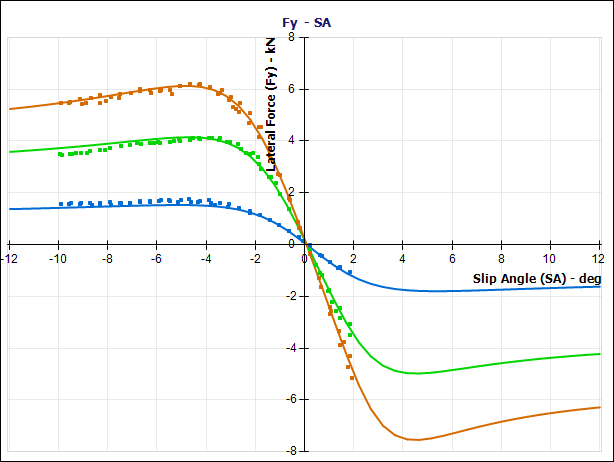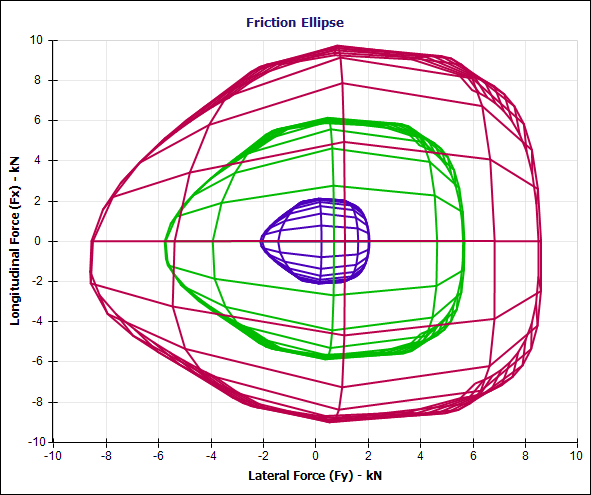### Asymmetric Tire Data and OptimumTire

Posted at April 10, 2012 | By : | Categories : Uncategorized | Comments Off

One issue our customers come across every now and then is an asymmetric tire data set. The data set itself is not really the issue, but it can complicate the process of fitting tire models using OptimumTire.

An asymmetric tire data set; is tire data collected on a tire testing machine (or vehicle) where parameters such as slip angle are not swept symmetrically around zero. The reason for limiting the test conditions like this can be many, for example: time/cost, tire wear, machine limitations or very specific test goals. If the data is to be used to fit semi-empirical tire models such as Pacejka/Magic Formula, it is beneficial to have data for as many tire states as possible. In the case of slip angle, it is easier to create a tire model which is representative of the real tire’s characteristics if we know its behavior for both slip angle directions.

In this example, where the slip angle is swept from -10° to 2°, it is difficult to know what the peak characteristics look like. OptimumTire will still fit a model to it, but we cannot be confident in the model around the peak for positive slip angles (around slip angle 5°).Data points from testing shown as dots, with the fitted Pacejka model overlaid. The different colors represent different vertical loads.

Another issue can be in combined testing (where we test both longitudinal and lateral forces at the same time). If we only have data for one slip angle direction (but both slip ratio directions), we might end up with something like this:Raw data from a combined tire test. The tire is tested at a multiple slip ratios and slip angles. But in this case only for one turn direction.

Similar to the first example, it will be difficult to know what the combined characteristics will look like for tire states where we don’t have data. A reasonable assumption in this case would be that the tire behaves symmetrically for both turn directions (unless we have information suggesting something else). If we want to use the tire models we create for vehicle simulation, we need to have a model that will give reasonable results in both turn directions.

If we stick with the assumption that the tire has similar combined characteristics for both turn directions, we can design a set of coefficient boundaries which will achieve this. We will want to lock the coefficients that cause asymmetry in the combined characteristics.  After reviewing the OptimumTire Help File, we can see how there are two coefficients that are of interest, rHx1 and rBy3. Both these coefficient introduces a shift. Based on the default coefficient boundaries in OptimumTire we create a new set of boundaries and we set rHx1 and rBy3 to zero and we set them to hard boundaries. This tells OptimumTire’s fitting algorithm that these coefficients must be zero, the algorithm will then search to find the best possible fit given these constraints.The coefficient boundaries selection menu in OptimumTire. By locking some coefficients to zero, we ensure symmetry.The longitudinal part of the combined model has been fit. The decrease in longitudinal force can be seen with increasing lateral force.

If we follow the procedure outlined in the OptimumTire Tutorials, we first fit the longitudinal parts of the model and then the lateral parts, in both cases using the new coefficient boundaries that we have created. We end up with something like this:Both longitudinal and lateral parts of the combined models have been fit. Good correlation between the data (dots) and model (lines) can be seen.

The result is a friction ellipse that is symmetric and matches the data well. Well, then, what does a non-symmetric friction ellipse look like? It can look something like this:An example of a highly asymmetric friction ellipse. Without data for both turn directions, it is difficult to know if this ellipse would be accurate or not.

Do you need tire data or tire models? OptimumG offers both consulting services and software that can help you with the process of acquiring tire data, fitting tire models and analyzing the results. Over the years, OptimumG have tested, modeled and analyzed different tires for tire manufacturers, vehicle manufacturers and race teams.